Courses

# Chapter Notes - Cost (Producer Behaviour and Supply), Class 12, Economics | EduRev Notes

## Commerce : Chapter Notes - Cost (Producer Behaviour and Supply), Class 12, Economics | EduRev Notes

The document Chapter Notes - Cost (Producer Behaviour and Supply), Class 12, Economics | EduRev Notes is a part of the Commerce Course Economics Class 12.
All you need of Commerce at this link: Commerce

COST

Cost of production : Expenditure incurred on various inputs to produce goods and services.

Cost function : Functional relationship between cost and output.

C=f(q)

Where        f=functional relationship

c= cost of production

q=quantity of product

Money cost : Money expenses incurred by a firm for producing a commodity or service.

Explicit cost : Actual payment made on hired factors of production. For example wages paid to the hired labourers, rent paid for hired accommodation, cost of raw material etc.

Implicit cost : Cost incurred on the self - owned factors of production.

For example, interest on owners capital, rent of own building, salary for the services of entrepreneur etc.

Opportunity cost : is the cost of next best alternative foregone / sacrificed.

Fixed cost : are the cost which are incurred on the fixed factors of production.

These costs remain fixed whatever may be the scale of output. These costs are present even when the output is zero.

These costs are present in short run but disappear in the long run.

Numerical example of fixed cost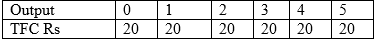TFC = Total Fixed Cost

Diagrammatic presentation of TFC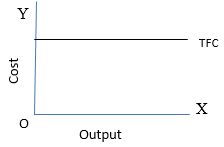TFC is also called as “overhead cost”, “supplementary cost”, and “unavoidable cost”.

Total Variable Cost: TVC or variable cost – are those costs which vary directly with the variation in the output. These costs are incurred on the variable factors of production.

These costs are also called “prime costs”, “Direct cost” or “avoidable cost”.

These costs are zero when output is zero.

Numerical example,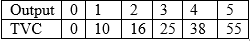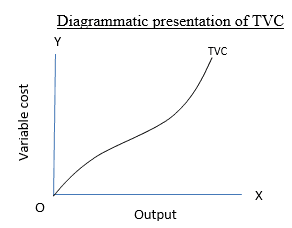Difference between TVC & TFC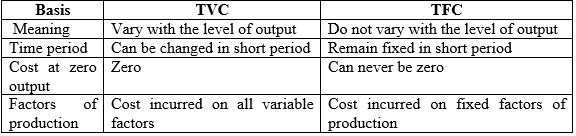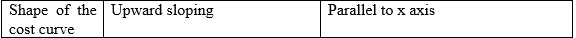Total cost : is the total expenditure incurred on the factors and non-factor inputs in the production of goods and services.

It is obtained by summing TFC and TVC at various levels of output.

Relation between TC, TFC and TVC

1. TFC is horizontal to x axis.
2. TC and TVC are S shaped (they rise initially at a decreasing rate, then at a constant rate & finally at an increasing rate) due to law of variable proportions.
3. At zero level of output TC is equal to TFC.
4. TC and TVC curves parallel to each other.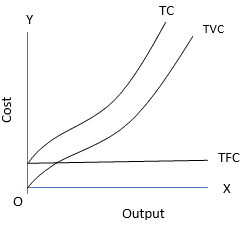• TC=TFC + TVC
• TFC=TC-TVC
• TVC=TC-TFC

Average cost : are the “cost per unit” of output produced.

Average fixed cost is the per unit fixed cost of production.

AFC = TFC / Q or output

AFC declines with every increase in output. It’s a rectangular hyperbola. It goes very close to x axis but never touches the x axis as TFC can never be zero.

Average variable cost is the cost per unit of the variable cost of production.

AVC = TVC / output.

AVC falls with every increase in output initially. Once the optimum level of output is reached AVC starts rising.

Average total cost (ATC) or Average cost (AC) : refers to the per unit total cost of production.

ATC = TC / Output

AC = AFC + AVC

Phases of AC

I phase : When both AFC and AVC fall , AC also fall

II phase : When AFC continue to fall , AVC remaining constant AC falls till it reaches minimum.

III phase : AC rises when rise in AVC is more than fall in AVC.

Important observations of AC , AVC & AFC

1. AC curve always lie above AVC (because AC includes AVC & AFC at all levels of output).
2. AVC reaches its minimum point at an output level lower than that of AC because when AVC is at its minimum AC is still falling because of fall in AFC.
3. As output increases, the gap between AC and AVC curves decreases but they never intersect.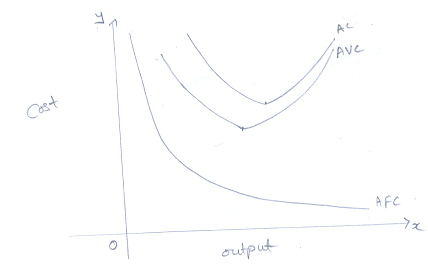Marginal cost : refers to the addition made to total cost when an additional unit of output is produced.

MC= TCn-TCn-1

MC = ΔTC / ΔQ

Note : MC is not affected by TFC.

Relationship between AC and MC

• Both AC & MC are derived from TC
• Both AC & MC are “U” shaped (Law of variable proportion)
• When AC is falling MC also falls & lies below AC curve.
• When AC is rising MC also rises & lies above AC
• MC cuts AC at its minimum where MC = AC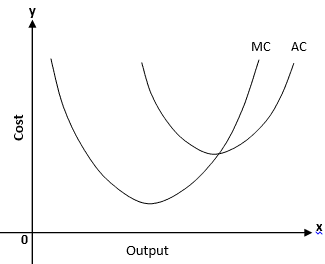Important formulae at a glance

1. TFC = TC – TVC or TFC=AFC x output or TFC = TC at 0 output.
2. TVC = TC – TFC or TVC = AVC x output or TVC =∑MC
3. TC = TVC + TFC or TC = AC x output or TC = ∑ MC + TFC
4. MCn=TCn – TCn-1 or MCn= TVCn – TVCn-1
5. AFC = TFC / Output or AFC = AC-AVC or ATC – AVC
6. AVC = TVC / Output or AVC = AC-AFC
7. AC = TC / Output or AC=AVC + AFC

Offer running on EduRev: Apply code STAYHOME200 to get INR 200 off on our premium plan EduRev Infinity!

## Economics Class 12

200 videos|214 docs|62 tests

,

,

,

,

,

,

,

,

,

,

,

,

,

,

,

,

,

,

,

,

,

,

,

,

,

,

,

;